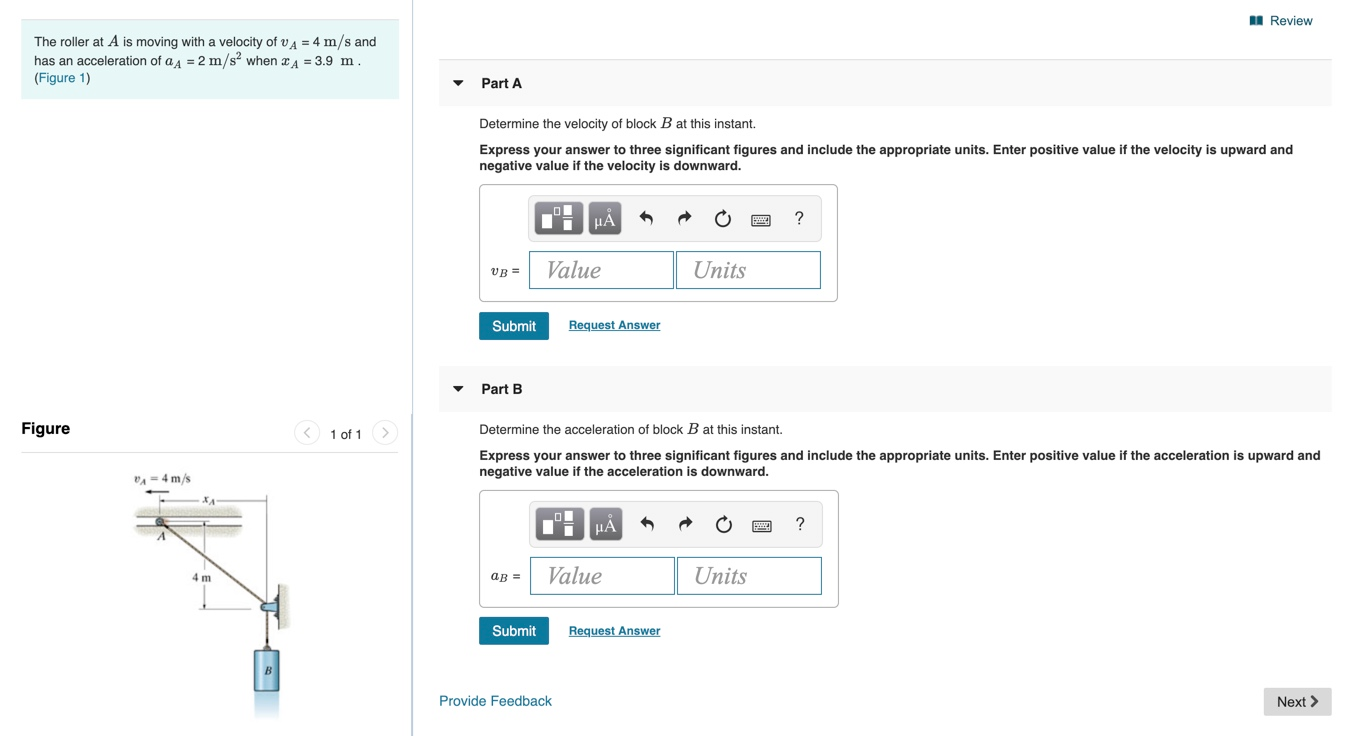Home / Answered Questions / Other / review-the-roller-at-a-is-moving-with-a-velocity-of-v-a-4-m-s-and-has-an-acceleration-of-a-a-2-m-s-w-aw254

# (Solved): Review The Roller At A Is Moving With A Velocity Of V A = 4 M/s And Has An Acceleration Of A A = 2 M...Review The roller at A is moving with a velocity of V A = 4 m/s and has an acceleration of a A = 2 m/s when 34 = 3.9 m. (Figure 1) Part A Determine the velocity of block B at this instant. Express your answer to three significant figures and include the appropriate units. Enter positive value if the velocity is upward and negative value if the velocity is downward. HÃ…R O 2 ? vb = Value Units Submit Request Answer Part B Figure < 1 of 1 Express your answer to three significant figures and include the appropriate units. Enter positive value if the acceleration is upward and negative value if the acceleration is downward. VA = 4 m/s 1: PÃ… O 2 ? ab = Value Units Submit Request Answer Provide Feedback Next >

We have an Answer from Expert